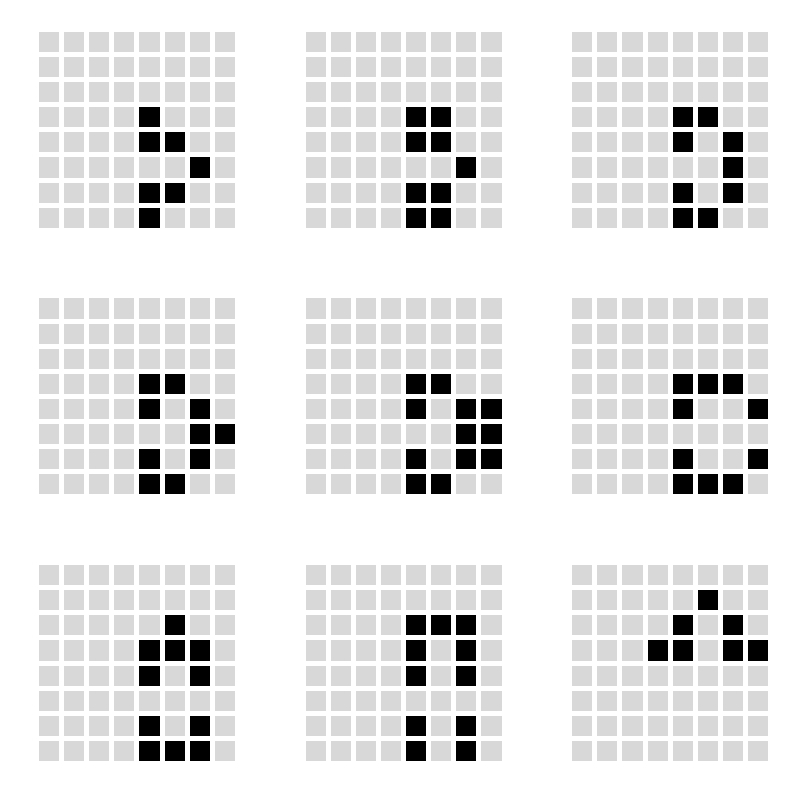# Looking For Life

` `

Machines take me by surprise with great frequency (Alan Turing)

Imagine a 8×8 grid in which cells can be alive (black colored) or empty (light gray colored):As with the One-dimensional Cellular Automata, the next state of a cell is a function of the states of the cell’s nearest neighbors (the only neighbors that we consider are the eight cells that form the immediate perimeter of a cell). To evolve this community of cells over time, rules are quite simple:

• If a live cell has less than two neighbors, then it dies (loneliness)
• If a live cell has more than three neighbors, then it dies (overcrowding)
• If an dead cell has three live neighbors, then it comes to life (reproduction)
• Otherwise, a cell stays as is (stasis)

These are the rules of the famous Conway’s Game Of Life, a cellular automaton invented in the late 1960s by John Conway trying to refine the description of a cellular automaton to the simplest one that could support universal computation. In 1970 Martin Gardner described Conway’s work in his Scientific American column. Gardner’s article inspired many people around the world to experiment with Conway’s, which eventually led to the final pieces of how the Game Of Life could support universal computation in what was surely a global collaborative effort.

In this experiment I will try to find interesting objects that can be found in the Game Of Life: static (remain the same over the time), periodic (change but repeating their initial configuration in some iterations) or moving objects (travel through the grid before disappearing). Why are interesting? Because these are the kind of objects required to perform computations.

The plan is simple: I will generate some random grids and will evolve them over time a significant number of times. After doing this, I will check those grids having still some alive cells inside. Will I find there what I am looking for?

I generated 81 grids in which live cells are randomly located using binomial random variables with probabilities equal to i/80 with i from 0 (all cells empty) to 80 (all cells alive). This is a quick way to try a set of populations with a wide range of live cells. I measure % of alive cells after each iteration. I will analyze those grids which still have live cells after all iterations. This is what happens after 150 iterations:I find some interesting objects. Since I keep them in my script, I can list them with `ls(pattern= "Conway", all.names = TRUE). `Two of them are specially interesting because are not static. Are those which produce non-constant lines in the previous plot.

First one is a periodic object which reproduces itself after every 3 iterations:Second one is a bit more complex. After 8 iterations appears rotated:What kind of calculations can be done with these objects? I don’t now yet but let’s give time to time. Do you want to look for Life?

```library(ggplot2)
library(scales)
library(gridExtra)
SumNeighbors = function (m) #Summarizes number of alive neighbors for each cell
{
rbind(m[-1,],0)+rbind(0,m[-nrow(m),])+cbind(m[,-1],0)+cbind(0,m[,-ncol(m)])+
cbind(rbind(m[-1,],0)[,-1],0)+cbind(0, rbind(0,m[-nrow(m),])[,-nrow(m)])+
cbind(0,rbind(m[-1,],0)[,-nrow(m)])+cbind(rbind(0,m[-nrow(m),])[,-1],0)
}
NextNeighborhood = function (m) #Calculates next state for each cell according to Conway's rules
{
(1-(SumNeighbors(m)<2 | SumNeighbors(m)>3)*1)-(SumNeighbors(m)==2 & m==0)*1
}
splits=80 #Number of different populations to simulate. Each population s initialized randomly
#according a binomial with probability i/splits with i from 0 to splits
iter=150
results=data.frame()
rm(list = ls(pattern = "conway")) #Remove previous solutions (don't worry!)
for (i in 0:splits)
{
z=matrix(rbinom(size=1, n=8^2, prob=i/splits), nrow=8); z0=z
results=rbind(results, c(i/splits, 0, sum(z)/(nrow(z)*ncol(z))))
for(j in 1:iter)
{z=NextNeighborhood(z); results=rbind(results, c(i/splits, j, sum(z)/(nrow(z)*ncol(z))))}
#Save interesting solutions
if (sum(z)/(nrow(z)*ncol(z))>0) assign(paste("Conway",format(i/splits, nsmall = 4), sep=""), z)
}
colnames(results)=c("start", "iter", "sparsity")
#Plot reults of simulation
opt1=theme(panel.background = element_rect(fill="gray85"),
panel.grid.minor = element_blank(),
panel.grid.major.x = element_blank(),
panel.grid.major.y = element_line(color="white", size=.5, linetype=2),
plot.title = element_text(size = 45, color="black"),
axis.title = element_text(size = 24, color="black"),
axis.text = element_text(size=20, color="black"),
axis.ticks = element_blank(),
axis.line = element_line(colour = "black", size=1))
ggplot(results, aes(iter, sparsity, group = start))+
geom_path(size=.8, alpha = 0.5, colour="black")+
scale_x_continuous("Iteration", expand = c(0, 0), limits=c(0, iter), breaks = seq(0,iter,10))+
scale_y_continuous("Alive Cells", labels = percent, expand = c(0, 0), limits=c(0, 1), breaks = seq(0, 1,.1))+
labs(title = "Conway's Game Of Life Simulation")+opt1
#List of all interesting solutions
ls(pattern= "Conway", all.names = TRUE)
#Example to plot the evolution of an interesting solution (in this case "Conway0.5500")
require(reshape)
opt=theme(legend.position="none",
panel.background = element_blank(),
panel.grid = element_blank(),
axis.ticks=element_blank(),
axis.title=element_blank(),
axis.text =element_blank())
p1=ggplot(melt(Conway0.5500), aes(x=X1, y=X2))+geom_tile(aes(fill=value), colour="white", lwd=2)+
scale_fill_gradientn(colours = c("gray85", "black"))+opt
p2=ggplot(melt(NextNeighborhood(Conway0.5500)), aes(x=X1, y=X2))+geom_tile(aes(fill=value), colour="white", lwd=2)+
scale_fill_gradientn(colours = c("gray85", "black"))+opt
p3=ggplot(melt(NextNeighborhood(NextNeighborhood(Conway0.5500))), aes(x=X1, y=X2))+geom_tile(aes(fill=value), colour="white", lwd=2)+
scale_fill_gradientn(colours = c("gray85", "black"))+opt
p4=ggplot(melt(NextNeighborhood(NextNeighborhood(NextNeighborhood(Conway0.5500)))), aes(x=X1, y=X2))+geom_tile(aes(fill=value), colour="white", lwd=2)+
scale_fill_gradientn(colours = c("gray85", "black"))+opt
#Arrange four plots in a 2x2 grid
grid.arrange(p1, p2, p3, p4, ncol=2)
```